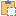#### SketchPlane Class

Represents a sketch plane or work plane.

Namespace: Autodesk.Revit.DB
Assembly: RevitAPI (in RevitAPI.dll) Version: 2015.0.0.0 (2015.0.0.0)

# Syntax

C#
``public class SketchPlane : Element``
Visual Basic
``````Public Class SketchPlane _
Inherits Element``````
Visual C++
``public ref class SketchPlane : public Element``

# Remarks

A SketchPlane object is used as an input to creation of sketch-referencing elements such as Model Curves or sketch-owning elements such as Generic Forms. The SketchPlane can be obtained from an existing element or created from a geometric plane or planar face. Note that the sketch plane element passed as input to create an element may not be the actual sketch plane assigned to that element; Revit may look for a geometrically equivalent plane to use, or may create a new one if the input plane is already used for other purposes. Some sketch planes (such as those obtained from detail curves) are suitable only for use in creating detail elements; they will be rejected when used for other element types.

# ExamplesCopy C#
``````public void ShowSketchPlane(SketchPlane sketchPlane)
{
// get the information of the created sketch plane
string information = "Sketch Plane: ";
//get the corresponding Plane
Autodesk.Revit.DB.Plane plane = sketchPlane.GetPlane();
//show the plane's origin point
information += "\norigin of the plane:  " + XYZToString(plane.Origin);
//show the plane's normal vector
information += "\nnormal of the plane:  " + XYZToString(plane.Normal);
//show the plane's Xvec vector
information += "\nXvec of the plane: (" + XYZToString(plane.XVec);
//show the plane's Yvec vector
information += "\nYvec of the plane: (" + XYZToString(plane.YVec);

}

// output the point's three coordinates
string XYZToString(XYZ point)
{
return "(" + point.X + ", " + point.Y + ", " + point.Z + ")";
}``````Copy VB.NET
``````Public Sub ShowSketchPlane(sketchPlane As SketchPlane)
' get the information of the created sketch plane
Dim information As String = "Sketch Plane: "
'get the corresponding Plane
Dim plane As Autodesk.Revit.DB.Plane = sketchPlane.GetPlane()
'show the plane's origin point
information += vbLf & "origin of the plane:  " & XYZToString(plane.Origin)
'show the plane's normal vector
information += vbLf & "normal of the plane:  " & XYZToString(plane.Normal)
'show the plane's Xvec vector
information += vbLf & "Xvec of the plane: (" & XYZToString(plane.XVec)
'show the plane's Yvec vector
information += vbLf & "Yvec of the plane: (" & XYZToString(plane.YVec)

End Sub

' output the point's three coordinates
Private Function XYZToString(point As XYZ) As String
Return "(" & Convert.ToString(point.X) & ", " & Convert.ToString(point.Y) & ", " & Convert.ToString(point.Z) & ")"
End Function``````

# Inheritance Hierarchy

System Object
Autodesk.Revit.DB Element
Autodesk.Revit.DB SketchPlane